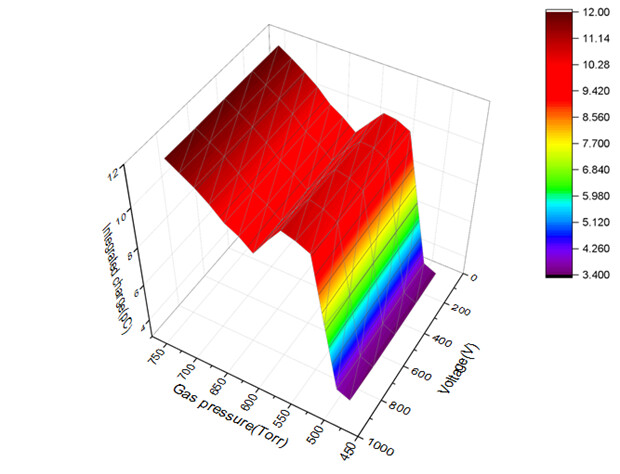# The relationship between the gas pressure, gas chamber voltage and integrated charge

The plot seems to show that the voltage dose not impact the integrated charge,

Yes, it shouldn’t (as we already discussed a couple of times).

Hi
You mean that the plot result is right?
Sorry, I still do not understand why the voltage has not impact on the integrated charge but do on the induced current.

If you wait long enough to collect all electrons and ions, the integral of the induced current corresponds to the deposited charge (as long as there is no multiplication happening). If you increase the voltage, the signal will be faster/shorter but the integral stays the same.

I only set 800 ns to collect the charge, the number of the electrons are 6 which is about half of the total electrons.

Besides, the range of the voltage is from 100 to 900 V, which is not only in the saturation region but also the proportional region.

Hi
I remember this plot in the book,

the X coordinate represents the voltage of the detector
the Y coordinate represents the electron/ion pairs collected
and the voltage is separated to five working region of the detector such as the saturation region, proportional region etc.

Yes, exactly.

Hi
So the plot I simulated is wrong,right?Why? You are in region “II” of the textbook plot you posted.

em…The voltage simulated is from 100V too 900V, but the region “||” is less than 200V . in the range of 200 to 800V is the proportional region.

I have no idea what gas is used in the textbook plot. The point is that as long as you don’t have multiplication, the integrated signal will be independent of the applied voltage. I think we have discussed this quite exhaustively now.

the gas is helium, So I should add the code about the multiplication at the proportional region, since the Garfield++ won’t simulate the multiplication problem automatic, right?

If you want to include multiplication you need to apply an electric field that is high enough for multiplication to occur (i. e. at which there is a non-zero Townsend coefficient) and you need to make sure that your gas file includes this range of electric fields.

This topic was automatically closed 14 days after the last reply. New replies are no longer allowed.